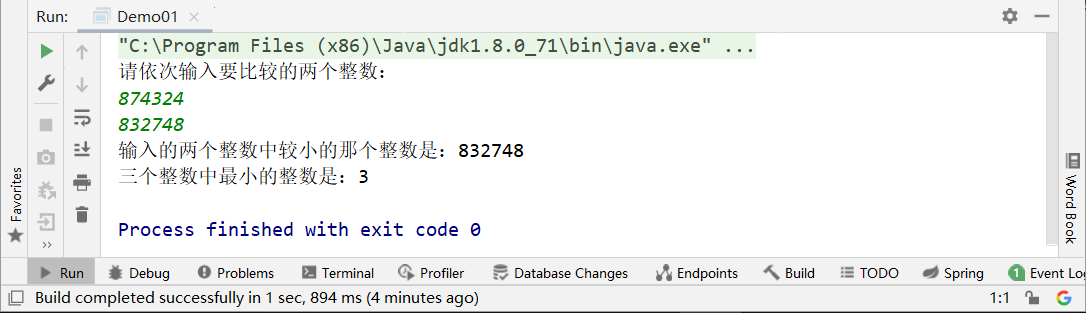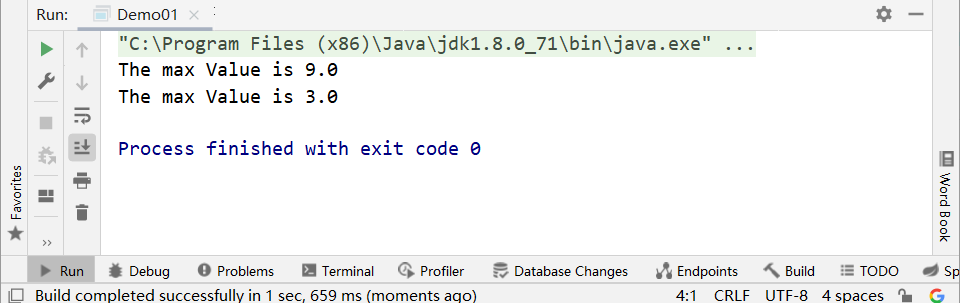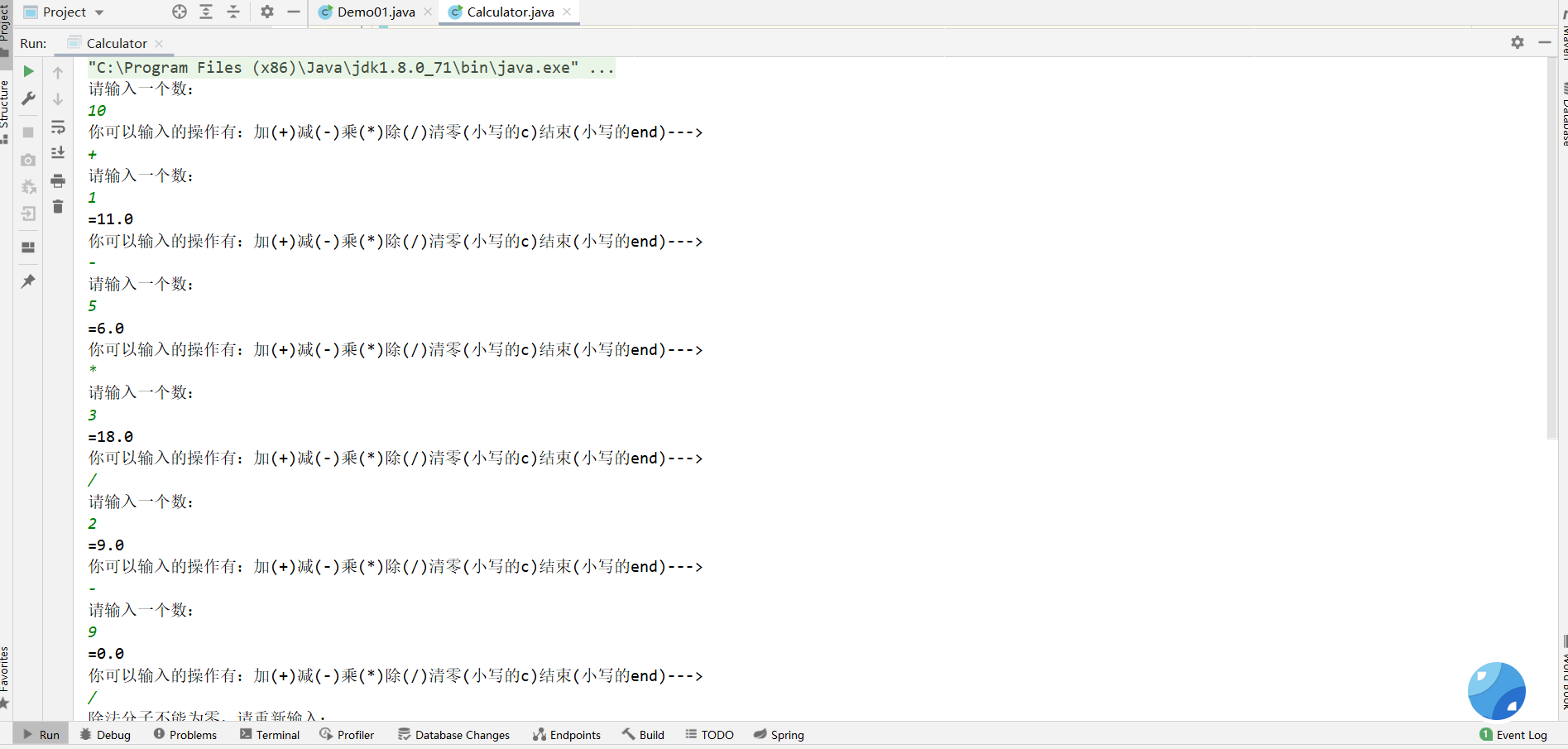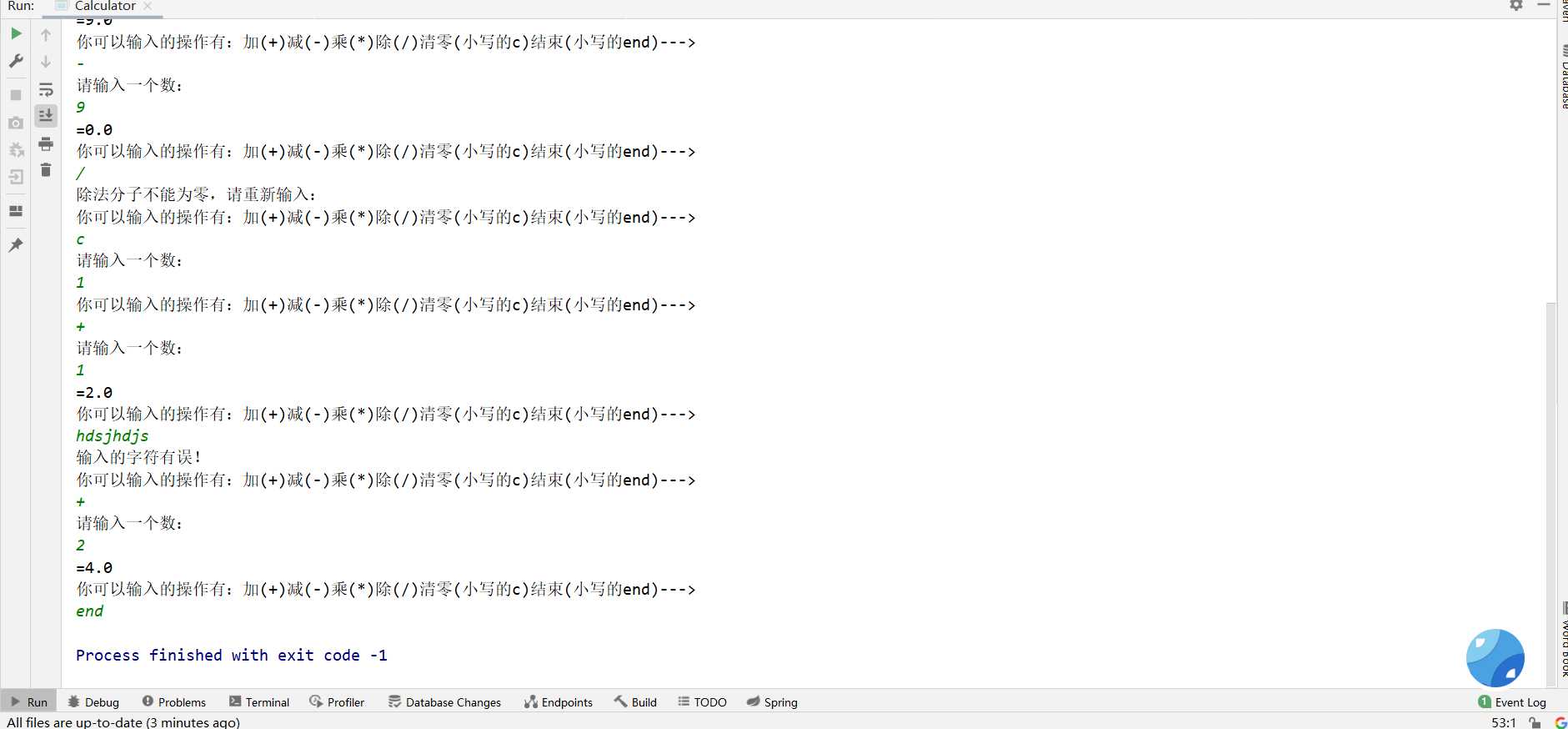# Java基础回顾_Java方法详解

## 何谓方法？

• Java方法是解决一类问题的步骤的有序组合
• 方法包含于类或对象中
• 方法在程序中被创建，在其它地方被引用

1. 方法命名遵循 小驼峰原则

2. main方法尽量保持简洁、干净

3. 方法其实就是C语言里的函数，方法遵循原子性，即一个方法只完成一件事

4. 有些方法没有return返回值，于是方法体就不需要写返回值类型，这个时候就要用 void 替代 返回值类型

5. 有参数的方法一定要有return返回值，没有参数的方法即void方法就不需要return返回值

6. 参数类型***这里得注意：参数类型分为***形式参数实参（或称为***变量***）。

形式参数：形参就是创建方法的时候我个人定义的像 int a, int b 这样的，没有实际值，等着调用的时候再往里传具体值的参数，相当于占位符，先把位置占着，用的时候再给它其他的值；

//执行的代码语句，即方法体

return + 返回值；

}

​ public int add( int a, int b ){

​ return a+b;

}

1. 有时候用return 0；去终止方法。
2. 方法的带你用：用对象名去点方法名，例如：对象名.方法名（实参列表）

***拓展了解：***值传递和引用传递

Java都是值传递！只有值传递！

## 方法的重载

``````public class Demo01 {
public static void main(String[] args) {
Scanner scanner = new Scanner(System.in);
System.out.println("请依次输入要比较的两个整数：");
int a = scanner.nextInt();
int b = scanner.nextInt();
int min = min(a, b);
System.out.println("输入的两个整数中较小的那个整数是："+min);
int min1 = min(6, 8, 3);
System.out.println("三个整数中最小的整数是："+min1);
}
public static int min(int a,int b){
int minNumber;
if (a<b){
minNumber = a;
}else {
minNumber = b;
}
return minNumber;
}
//重载的取最小值的方法，但是参数列表不一样，多了一个参数
public static int min(int a,int b,int c){
int minNumber;
if (a<b){
minNumber = a;
}else {
minNumber = b;
}
if(minNumber<c){
return minNumber;
}else {
minNumber=c;
}
return minNumber;
}
}
``````1. 方法名称必须一模一样
2. 参数列表必须不同，即参数的***个数不同***、或参数的***类型不同***、或参数的***排列顺序不同***等
3. 方法的***返回值类型可以相同，也可以不相同***，但是仅仅只有返回值类型不同也不行

## 命令行传参（不经常使用）

• 有时候你希望运行一个程序的时候，再传递给它消息。这个时候就要靠传递命令行参数给main()函数去实现。

## 可变参数

``````public class Demo01 {
public static void main(String[] args) {
printMax(1,5,3,4,6,8,2,3,9,6,4);
printMax(new double[]{1,2,3});
}
public static void printMax(double... numbers){
if (numbers.length == 0){
System.out.println("No argument passed");
return;
}
double result = numbers;

//排序！
for (int i = 1; i < numbers.length; i++) {
if (numbers[i] > result){
result = numbers[i];
}
}
System.out.println("The max Value is " + result);
}
}
``````## 递归（重点）

1. 递归头： 就是有一串可以停止调用方法（函数）的 代码，让程序不会无限循环运行！
2. 递归体：

``````import java.util.Scanner;

public class Calculator{
public static void main(String[] args) {
//创建对象用来调用对象里的方法
Calculator calculator = new Calculator();
//调用computer方法
calculator.computer();
}
//第一步：加减乘除四个方法（因为要循环计算，每次传入一个数字，所以参数列表应该只有一个参数）
//加法
System.out.println("请输入一个数：");
Scanner scanner3 = new Scanner(System.in);
double b = scanner3.nextDouble();
return a+b;
}
//减法
public static double subtract(double a){
System.out.println("请输入一个数：");
Scanner scanner3 = new Scanner(System.in);
double b = scanner3.nextDouble();
return a-b;
}
//乘法
public static double multiply(double a){
System.out.println("请输入一个数：");
Scanner scanner3 = new Scanner(System.in);
double b = scanner3.nextDouble();
return a*b;
}
//除法
public static double divide(double a){
System.out.println("请输入一个数：");
Scanner scanner3 = new Scanner(System.in);
double b = scanner3.nextDouble();
return a/b;
}

//第二步：完成循环输入数据的功能（while（true）+switch）,必须创建一个方法完成这一步
public void computer(){
System.out.println("请输入一个数：");
Scanner scanner1 = new Scanner(System.in);
double a = scanner1.nextDouble();

while(true){
Scanner scanner2 = new Scanner(System.in);
System.out.println("你可以输入的操作有：加(+)减(-)乘(*)除(/)清零(小写的c)结束(小写的end)--->");
String str = scanner2.nextLine();
switch (str){
case "+":
System.out.println("="+a);
break;
case "-":
a = subtract(a);
System.out.println("="+a);
break;
case "*":
a = multiply(a);
System.out.println("="+a);
break;
case "/":
if (a == 0){
System.out.println("除法分子不能为零，请重新输入：");
break;
}
a = divide(a);
System.out.println("="+a);
break;
case "c":
computer();
break;
case "end":
System.exit(-1);
break;
default:
System.out.println("输入的字符有误！");
}
}
}

}
``````